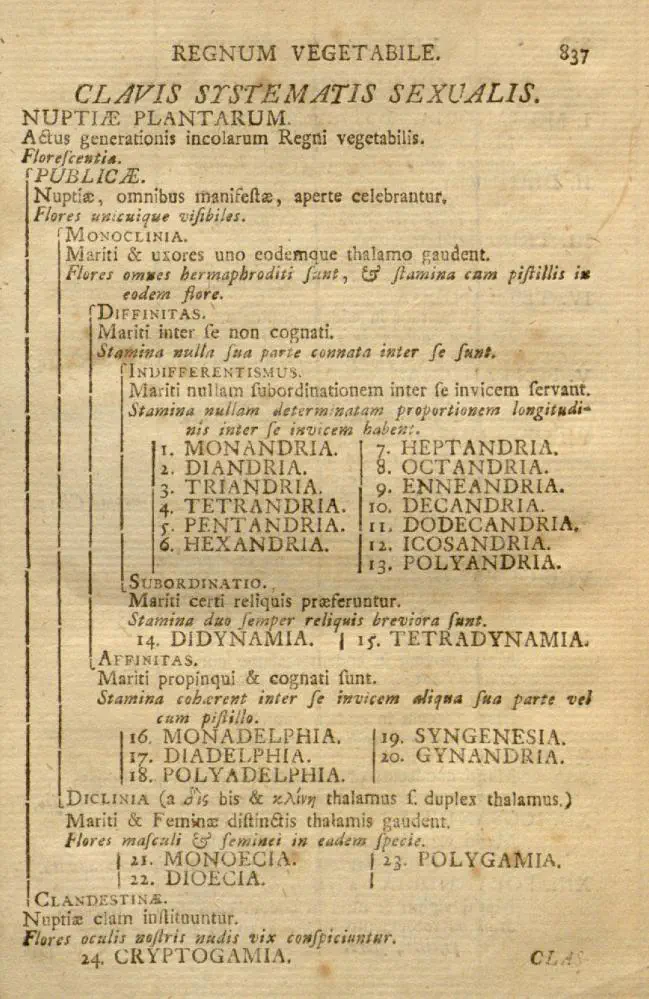# Communication Complexity and Quantum Optimization Lower Bounds via Query ComplexityOne of the first published decision trees

### Abstract

My PhD work was in the areas of query and communication complexity. These complexity models have a rich background connecting them to each other, to polynomials, to matrices, and they also serve as practical abstractions for various computing paradigms. In this thesis we provide more insight into these connections. The work in this thesis comes from three published papers:

• The Log-Approximate-Rank Conjecture Is False, with Arkadev Chattopadhyay and Nikhil S. Mande: There are numerous neat connections between communication protocols and matrix ranks/norms. We show that certain conjectured connections between randomized communication protocols and approximate rank/matrix norms do not hold.
• Towards Stronger Counterexamples to the Log-Approximate-Rank Conjecture, with Arkadev Chattopadhyay and Ankit Garg: We look at some simple functions arising from a combinatorial structure known as subspace designs. We show evidence that these simple functions are hard to compute. The simplicity of the functions and the conjectured hardness are even more glaringly at odds with each other than in the function presented in the previous work. We put forth a fundamental conjecture about subspaces that would prove the conjectured hardness if true.
• No Quantum Speedup over Gradient Descent for Non-Smooth Convex Optimization, with Ankit Garg, Robin Kothari and Praneeth Netrapalli: Optimization algorithms are often black-box algorithms, looking at the outputs of the functions they are trying to optimize rather than looking at the inner workings of the function. This abstraction of a black-box algorithm is captured by query complexity. The well-known Gradient Descent is a black-box algorithm that is provably optimal for the task of non-smooth convex optimization. Using known techniques in quantum query complexity, we are able to show that Gradient Descent can not be improved upon by a quantum black-box algorithm. This is in contrast to other quantum black-box algorithms such as Grover’s search and Shor’s period finding algorithm which show significant speedups.

• Conjecture 7.3 is false! The binary NAND tree with $n$ leaves is a function that has $\Omega(n)$ subspace decision tree depth (witnessed by a lower bound of $2^{\Omega(n)}$ on the $L_1$ norm of the Fourier coefficients of $f$), but has a randomized protocol (not even using parities) that is polynomially more efficient (see for instance Probabilistic boolean decision trees and the complexity of evaluating game trees, Saks and Wigderson ‘86 [Example 1.1]). Thanks to Swagato Sanyal for pointing this out.
• Conjecture 7.1 is true! TsunMing Cheung, Hamed Hatami, Kaave Hosseini and Morgan Shirley proved in Separation of the factorization norm and randomized communication complexity that the $\gamma_2$ norm of the Integer Inner Product function is large despite it being easy to compute using randomized communication.